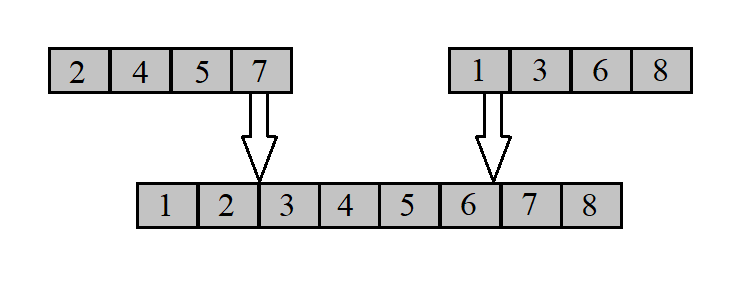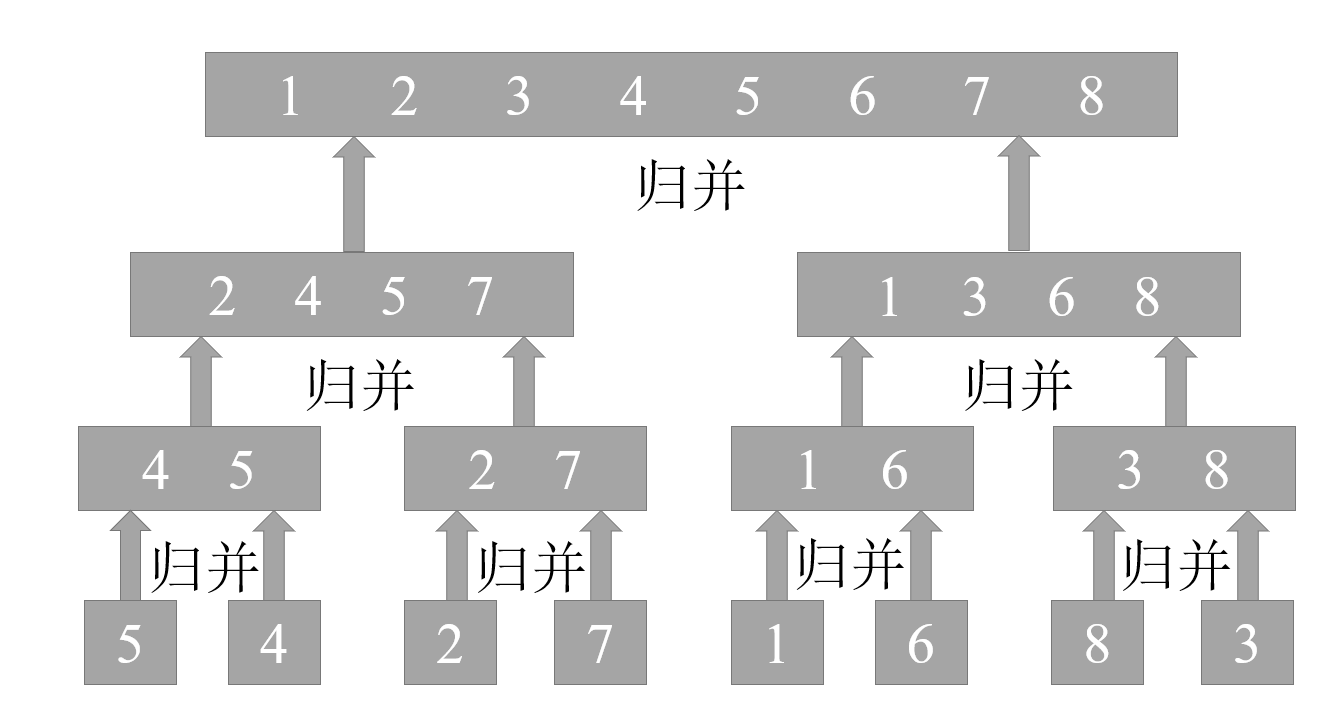# 算法与数据结构（2）：时间复杂度——以归并排序为例

2019/04/10 10:10

### 归并排序#### 归并排序的合并步骤

// 合并两个相邻的数组
// 将已经有序的数组 array[0, array_length1 - 1] 与 array[array_length1, array_length1 + array_length2 - 1]
// 合并至 array[0, array_length1 + array_length2 - 1]，并依然保证有序
int Merge(int* array, int array_length1, int array_length2){
int i, j, k; // 临时变量
int* temp_array1 = (int*)malloc(sizeof(int) * (array_length1 + 1));
int* temp_array2 = (int*)malloc(sizeof(int) * (array_length2 + 1));

// 复制新数组，因为原数组将会用于储存结果
for(i = 0; i < array_length1; i++){
temp_array1[i] = array[i];
}
temp_array1[array_length1] = INT_MAX;
for(i = 0; i < array_length2; i++){
temp_array2[i] = array[array_length1 + i];
}
temp_array2[array_length2] = INT_MAX;

// 进行合并操作
j = 0;
k = 0;
for(i = 0; i < array_length1 + array_length2; i++){ // 我是第22行
if(temp_array1[j] > temp_array2[k]){
array[i] = temp_array2[k];
k++;
} else{
array[i] = temp_array1[j];
j++;
}
}

// 释放申请的空间
free(temp_array1);
free(temp_array2);
return 0;
}#### 归并排序的其他步骤

// 归并排序，结果按升序排列
int MergeSort(int* array, int array_length){
// 若数组长度为1，必然有序
if(array_length == 1){
return 0;
}

int half_array_length = array_length / 2;
// 将数组分为两个部分，递归实现子数组的排序
MergeSort(array, half_array_length);
MergeSort(array + half_array_length, array_length - half_array_length);
// 将已经排序完成的子数组合并，实现整个数组的排序
Merge(array, half_array_length, array_length - half_array_length);

return 0;
}#### 归并排序的运行效率分析

T(n)表示规模为n的一个问题的运行时间。当问题规模足够小的时候，直接求解仅需要常量时间，如归并排序时数组长度为1时，记为$\Theta (1)$，含义与数学中渐进分析一样，我们通俗的理解为等于某个常数，规范解释我们将在下文的时间复杂度中详细介绍。

### 时间复杂度

#### 渐进符号

$\Theta$ 记号我们前面已经看到过了，读作 Theta ，在这里，我们给出他的一个详细定义：

### 结语### 作者的其它热门文章

0
0 收藏

0 评论
0 收藏
0